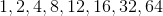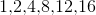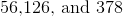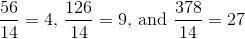## Example Questions

### Example Question #1 : Factors / Multiples

What is the greatest common factor of 49, 91, and 119?

7

1

3

9

7

Explanation:

The greatest common factor is the largest integer that divides without remainder into a set of integers. In this case, 1 and 7 are both common factors, but 7 is the greatest common factor.

### Example Question #2 : Greatest Common Factor

What is the greatest common factor of 55, 165, and 220?

5

11

20

55

55

Explanation:

The greatest common factor is the largest factor that the given numbers have in common. 5 is a common factor, but it is not the greatest one; 11 and 20 are not common factors to the given numbers; however, 55 is a common factor of the given three numbers and is the greatest one at that.

### Example Question #1 : How To Find The Greatest Common Factor

What is the greatest common factor of 30, 90, and 120?

15

30

25

3

10

30

Explanation:

The greatest common factor can most easily be found by plugging in answers from your answer choices, a good strategy with these types of problems is to simply guess and check using your largest answer, in this case, we see that 30 is immediately the GCF for these three numbers

### Example Question #1641 : Act Math

What is the GCF of 36, 64, and 144?

2

6

4

36

12

4

Explanation:

GCF=greatest common factor. First find the factors of each number. So the factors for each number are

36: 1, 36, 2, 18, 3, 12, 4, 9, 6

64: 1, 64, 2, 32, 4, 16

144:1, 144, 2, 72, 3, 48, 4, 36, 6, 24, 8, 18, 9, 16, 12.

1, 2, and 4 are the only factors that are common to 36, 64, and 144. Since 4 is the greatest of these factors, it is the correct answer.

### Example Question #1642 : Act Math

What is the greatest common factor of 36,108, and 180?

5

2

3

18

36

36

Explanation:

We can find the greatest common factor of a series of numbers by performing the prime factorization of each number. The greatest common factor will be the product of the combinations of prime factors that each number has in common. 36 can be factorized to 2* 32.108 can be factorized to 2* 33.  180 can be factorized to 3* 2* 5. The greatest common prime factorization is 2* 32, the product of which is 36. So 36 is the greatest common factor. Remember that 2* 3= 2* 3* 3.

### Example Question #1 : How To Find The Greatest Common Factor

What is the greatest common factor of 56 and 68?

8

14

4

7

4

Explanation:

The factors are as follows:

56: 1,2,4,7,8,14,28,56

68, 1,2,4,17,34,68

The greatest factor that both numbers are divisible by is 4.

### Example Question #1 : How To Find The Greatest Common Factor

What is the greatest common factor of 72 and 84?

10

18

6

12

12

Explanation:

Factors are numbers that can divide evenly into larger numbers. The factors of 72 are 36, 24, 18, 12, 9, 8, 6, 4, 3, and 2. The factors of 84 are 42, 28, 21, 14, 12, 7, 6, 4, 3, and 2. Thefore, the greatest common factor is 12.

### Example Question #1 : Greatest Common Factor

What is the greatest common factor (GCF) of 48, 156, and 420?

12

4

24

6

2

12

Explanation:

All three numbers are divisible by 2, 4, 6, and 12, but only 48 is divislbe by 24. Therefore, the greatest common factor of these three numbers is 12.

### Example Question #1 : How To Find The Greatest Common Factor

What is the greatest common factor ofand?Explanation:

The greatest common factor is the largest factor that goes into both numbers. Begin by factoring each number:.

The common facors are,, and the greatest of these is### Example Question #5 : How To Find The Greatest Common Factor

What is the greatest common factor of?Explanation:

There are two approaches to this question. You can either list all of the factors for each of the numbers given in the question and see which one is the greatest that the three have in common. The other approach is to check each of the three numbers in the question by the answer choices and see which is the greatest factor of all three numbers.

In this case:Note thatis a common factor of these numbers, but is obviously smaller than.

### All ACT Math Resources